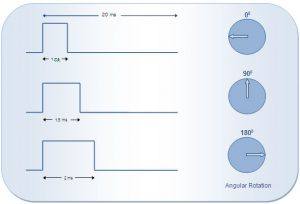# How to interface Servo Motor with PIC Microcontroller- (Part 21/25)

Servo systems use the error sensing negative feedback method to provide precise angular motion. Servo Motors are used where precise control on angular motion is needed. Servo motors are widely used in the field of Robotics to design robotic arms, palms, legs and so on. They are also used in RC toys like RC helicopter, airplanes and cars.

The interfacing of servo motor using PIC microcontroller has been explained here. Readers are advised to go through the article on Servo Motors to learn basic mechanism and control of servo motor.

Servo motor has three wire terminals : two of these wires are to provide ground and positive supply to the Servo DC motor, while the third wire is for the control signal. These wires of a servo motor are color coded. The servo motor can be driven only when PWM (pulse width modulated) signals are provided to the control terminal.

The total pulse duration for a typical servo motor should be of 20 milliseconds. The on-time duration of the control signal varies from 1ms to 2ms. This on-time variation provides angular variation from 0 to 180 degree. Also refer Servo motor control using 8051.According to the above diagram, desired angular position can be calculated by simple interpolations. For example, if the servo motor should be positioned at 45° angle, the desired output control pulse can be obtained as follows:

180° angular displacement is achieved by the pulse duration = 1 ms
1° angular displacement is achieved by the pulse duration of = 1 /180 ms
45° angular displacement is achieved by the pulse duration of = (1/180) x 45 = 0.25 ms
So total on-time pulse will be = 1ms + 0.25ms =1.25 ms

Please note that the on-time duration of the control signal may vary based on the manufacturer or certain other conditions. Therefore it is imperative that the on-time pulse for 0° and 180° positions must be obtained (either from the datasheet or by hit-n-trial) before using a servo motor for an application. The servo motor used here moves to 0° at 0.55 ms pulse.

Objective: To interface the servo motor with PIC18F4550 microcontroller and generate pulses to rotate the servo spline in step angles (of 45°) from 0° to 180°. Please check the Video tab to see these rotations. After reaching 180° position, the spline is brought back to 0° position and the rotation thus continues.

Programming Steps:

1.         Calculate the on-time duration for 1° angular displacement.
2.         Create delay function to calculate time duration.
3.         Set the pin high at which control terminal of the servo motor is connected.
4.         Call the delay function (mentioned in 3) and repeat it number of times as much angular displacement needed.
5.         Set the control pin low.
6.         Generate delay of 18 ms.
7.         Repeat the steps from 4 to 7 continuously for about 50 times to send a train of pulses.

### Project Source Code

###

READ  Basic Servo Motor Controlling with Microchip PIC Microcontroller

// Program to control Servo angular position using PIC18F4550 Microcontroller
// The on-time pulse for 0° position has been taken as 0.55ms
// Delay_ms() provides delay in milliseconds while Delay_us() provides delay in microseconds

unsigned int angle=0,i;
void cs_delay(unsigned int);
void main()
{
TRISB.F0=0;
while(1)
{
for(angle=0;angle<=180;angle+=45) // Loop to increase the angle by 45°
{
for(i=0;i<50;i++) // Loop to provide continuous train of pulse
{
LATB.F0=1; // Send high to control signal terninal
cs_delay(angle); // Call delay function
LATB.F0=0; // Send low to control signal terninal
Delay_ms(18);
}
}
}
}

void cs_delay(unsigned int count)
{
int j=0;
Delay_us(550); // Delay to move the servo at 0°
for(j=0;j<count;j++) // Repeat the loop equal to as much as angle
{
Delay_us(6); // Delay to displace servo by 1°
}
}

###

### Circuit Diagrams### Project Video